# 这个递归不太难

## 一、先热个身

``function sum(n) {     let count = 0;     for (let i = 1; i <= n; i++) {     	count += i;     }     return count; } sum(100); // 5050 ``

``count += i; ``

``function sum(n) {     return n + sum(n-1); } // 这里的 sum(n-1) 不能写成 sum(n--) ``

``function sum(n) { 		if (n === 1) { 			return n; 		}     return n + sum(n-1); } sum(100); // 5050 ``

## 二、三大要素

1. 递归的拆解 —— 提取重复的逻辑，缩小问题规模
2. 递归的出口 —— 明确递归的结束条件

1. 递归的定义 —— 明确函数的功能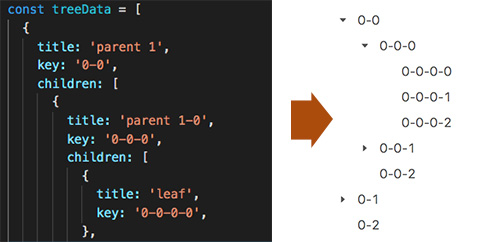``renderTree = (list, level = 1) => {   return list.map(x => {     const { children, id } = x || {};     if (children) { // 递归的结束条件       return (         <TreeNode key={`\${id}`} level={level}>           {/* 调用自身，形成递归 */}           {renderTreeNodes(children, level + 1)}         </TreeNode>       );     }     // 递归的出口     return <TreeNode key={`\${id}`} level={level}></TreeNode>   }) } ``

## 三、递归优化 – 手动缓存

``function Fibonacci (n) {   return n <= 2 ? 1 : Fibonacci(n - 1) + Fibonacci(n - 2); } ``

Fibonacci 数列：1, 1, 2, 3, 5, 8, 13, 21…

f(n) = f(n-1) + f(n-2)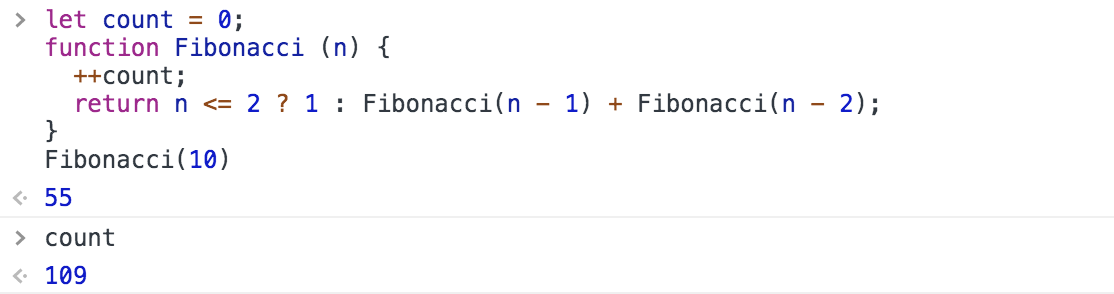``-- f(5) | -- f(4) | | -- f(3) | | | -- f(2) | | | -- f(1) | | -- f(2) | -- f(3) | | -- f(2) | | -- f(1) ``

``// 缓存函数 function memozi(fn){ 	const obj = {};   return function(n){     obj[n] = obj[n] || fn(n);     return obj[n];   } }  const Fibonacci = memozi(function(n) {   return n <= 2 ? 1 : Fibonacci(n - 1) + Fibonacci(n - 2); }) ``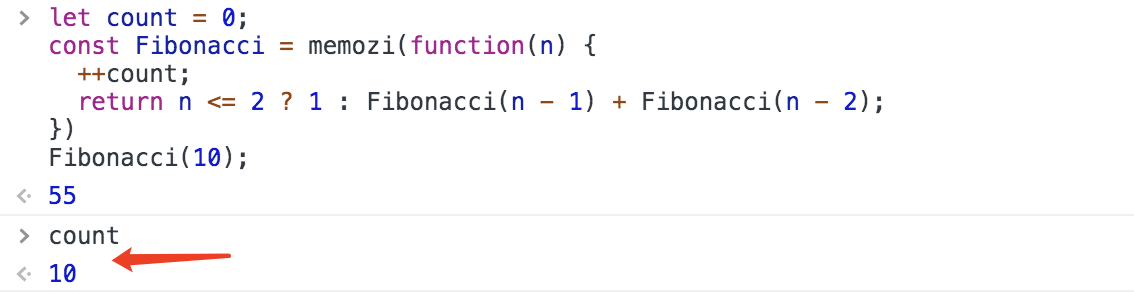## 四、递归优化 – 自下而上

``function foo(x = 1, y = 1) {   return foo(y, x + y); } ``

``function Fibonacci(n, x = 1, y = 1) {   return n <= 2 ? y : Fibonacci(n - 1, y, x + y); } ``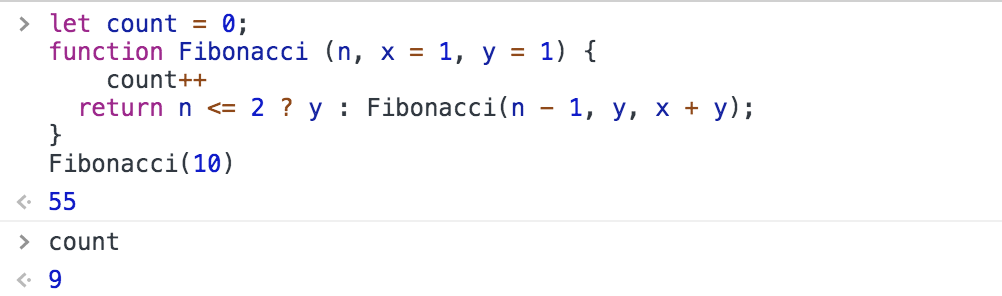1. 最优子结构
2. 边界

1. 状态转移方程

1. 存在最优子结构

作为整个过程的最优策略，应当具有这样的特质：无论过去的状态和决策如何，相对于前面的决策所形成的状态而言，余下的决策序列必然构成最优子策略。

也就是说，一个最优策略的子策略也是最优的。

2. 无后效性

如果某阶段状态给定后，则在这个阶段以后过程的发展不受这个阶段以前各段状态的影响。

也就是说，计算`f(i)`，不需要`f(i+1)...f(n)`的值，也不会修改`f(1)...f(i-1)`的值（1 < i < n）。

## 五、递归优化 – 尾调用

``function foo () { console.log('wise'); } function bar () { foo(); } function baz () { bar(); }  baz(); ``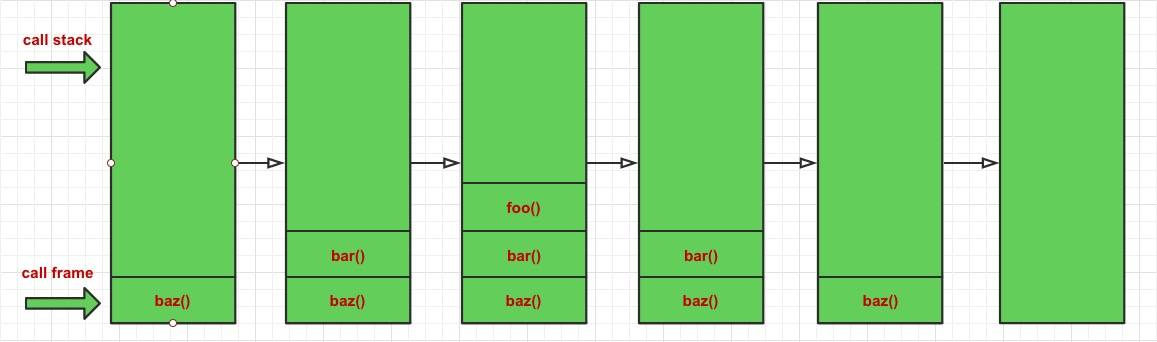``function foo () { console.log('wise'); } function bar () { return foo(); } function baz () { return bar(); }  baz(); ``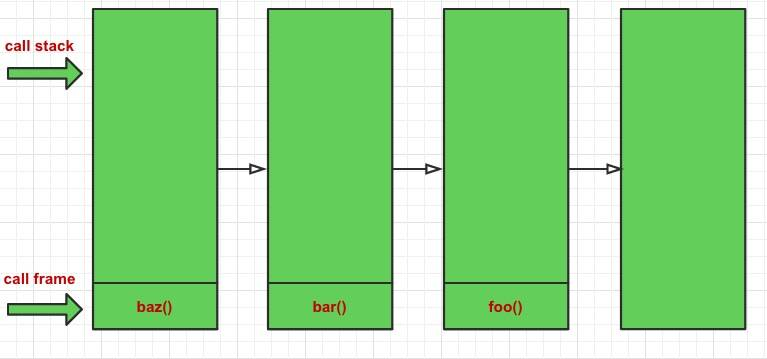``function sum(n) { 		if (n === 1) { 			return n; 		}     return n + sum(n-1); } sum(100); // 5050 ``

``Uncaught RangeError: Maximum call stack size exceeded ``

``function sum(n, count = 0) { 		if (n === 1) { 			return count + n; 		}     return sum(n-1, count+n); } ``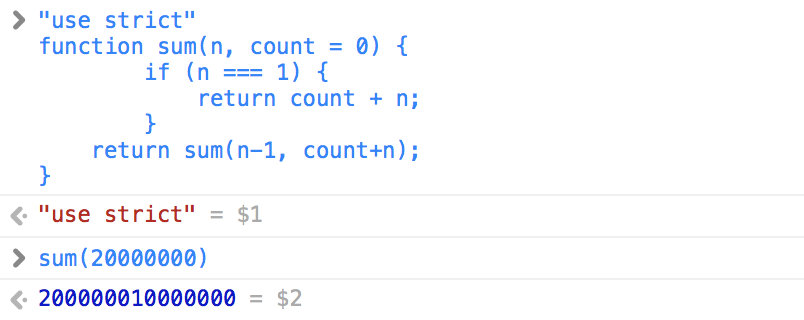Chrome V8 团队给出的解释是：

1. 由于尾调用消除调用帧是隐式的，这意味着开发者可能很难发现一些无限循环的递归，如果它们恰好出现在末尾，因为这些递归的堆栈将不再溢出。
2. 尾调用会丢失堆栈信息，这将导致执行流中的堆栈信息丢失，这将影响程序调试和错误收集。

## 六、小结

1. 递归的优点是代码简洁，逻辑清晰。缺点是调用帧导致的执行效率过低，而且不如循环容易理解；
2. 递归和循环完全可以互换，但递归可以处理的问题，如果通过循环去解决，通常需要额外的低效处理；
3. 如果逻辑相对简单，使用循环也很简洁，可以优先考虑循环；
4. 在无法使用尾递归的环境，循环永远是优先考虑的解决方案，但如果能接受递归的性能开销，建议使用递归。

Premature optimization is the root of all evil.

—— 《计算机编程艺术》Donald Knuth

### 参考资料：

《递归优化：尾调用和Memoization》—— LumiereXyloto

《尾调用优化》—— 阮一峰

《【译】V8 团队眼中的 ES6、ES7及未来》—— 奇舞团

《什么是动态规划（Dynamic Programming）？动态规划的意义是什么？》—— 苗华栋

Wise.Wrong资料部分资料来自网络，侵权毕设源码联系删除

qklbishe.com区块链毕设代做网专注|以太坊fabric-计算机|java|毕业设计|代做平台 » Wise.Wrong资料